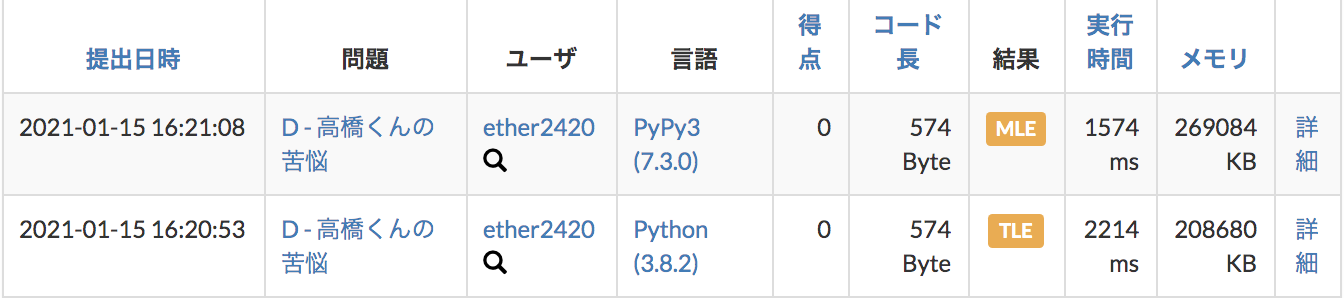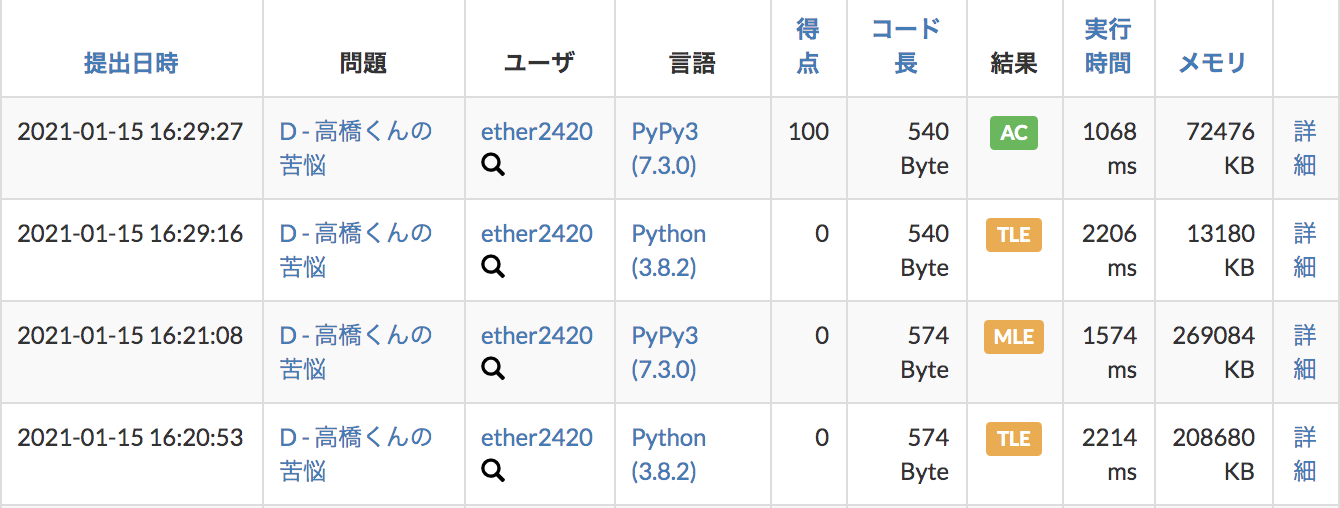# [Python] Technique to reduce dimensions with DP (Dynamic Programming) [AtCoder]

Recently I started studying DP ** Techniques to reduce the dimension of DP ** I was very impressed with it, so I will write an article! !! !!

I would like to briefly explain the following two questions while actually solving them.

-TDPC A-Contest ** → DP from 2D to 1D ** -ABC 015 D --Takahashi-kun's suffering ** → DP from 3D to 2D **

# TDPC A --Contest

This is a typical subsum problem. The problem this time is that MAX of N is 100 → Bit full search is impossible in terms of computational complexity, so consider it in DP.

## DP: 2D Ver

Normally, when you solve DP as two dimensions, it looks like this

• Note that the score pattern is 0 to ** MAX10000 ** (100 * 100) points, not 0 to 100 points.

#### `DP_2D.py`

``````
import itertools,sys
#############################
N = I()
p = LI()

dp = [*10001 for _ in range(N+1)] #0:That score does not exist, 1:That score exists
dp = 1
for i,j in itertools.product(range(1,N+1),range(10001)):
pi = p[i-1]
if j-pi>=0:
dp[i][j] = max(dp[i][j],dp[i-1][j-pi]) #If you choose
dp[i][j] = max(dp[i][j],dp[i-1][j])        #If you do not choose

print(sum(dp[-1])) #Output the number of numbers 1
``````

## DP: 1D Ver

To make this DP1 dimension, do this!

#### `DP_1D.py`

``````
import itertools,sys
#############################
N = I()
p = LI()

dp = *10001 #0:That score does not exist, 1:That score exists
dp = 1
for i,j in itertools.product(range(1,N+1),range(10001)[::-1]):
pi = p[i-1]
if j-pi>=0:
dp[j] = max(dp[j],dp[j-pi]) #If you choose
#dp[j] = max(dp[j],dp[j])       #If not selected → Not required

print(sum(dp)) #Output the number of numbers 1
``````

** The point is here! !! !! ** ** `range(10001)[::-1]`

• `Range (10000, -1, -1)` is also acceptable

** You can reuse dp by turning the loop from the opposite side! !! !! !! !! This is the technique! !! !! !! !! ** **

If you use dp and turn the loop in order For example, when p = 2 points,

dp = [1,0,1,0,1,0,1,0,1 ・ ・ ・]

And 4 points, 6 points, 8 points ... will also exist ... Because `dp[j] = max(dp[j],dp[j-pi])` Since 2 points are 1, 4 points are also 1. .. .. Since 4 points are 1, 6 points are also 1. .. .. ・ ・ ・

** But if you loop from the opposite ... **

dp = [1,0,1,0,0,0,0,0,0 ・ ・ ・]

`dp[j] = max(dp[j],dp[j-pi])`

・ ・ ・ Since 4 points are 0, 6 points will also be 0! Since 2 points are 0, 4 points will also be 0! Since 0 points are 1, 2 points become 1! !! !! ** So you can get the correct result while using dp around! !! !! !! !! !! !! !! !! → You can reduce the dimension of dp from 2D to 1D! !! !! !! !! !! !! !! !! !! ** **

** By lowering the dimension of dp, I'm so happy that the amount of thinking in my head has decreased! ** **

I wonder if this impression can be transmitted ~ ** Please tell me ~ **

Another question to make you feel more impressed! !! !!

# ABC 015 D --Takahashi-kun's Anguish

Difficulty: 1388 light blue problem! A slightly more advanced problem with one more variable in the knapsack DP.

## DP: 3D Ver

If you think about DP in 3D, it's not impossible, but my head seems to be punk ...

#### `DP_3D.py`

``````
import itertools,sys
#############################
W = I()
N,K = LI()
AB = [LI() for _ in range(N)]

dp = [[*(W+1) for _ in range(K+1)] for _ in range(N)] #Holds the maximum value of importance when using k sheets
for i,k,w in itertools.product(range(N),range(1,K+1),range(W+1)):
A,B = AB[i]
if w-A>=0:
dp[i][k][w] = max(dp[i][k][w],dp[i-1][k-1][w-A]+B)
dp[i][k][w] = max(dp[i][k][w],dp[i-1][k][w])

print(dp[-1][-1][-1])
``````

However, with this, ** Python is computationally TLE, and PyPy is MLE **. Owata ...## DP: 2D Ver

**here! Using the dimensionality reduction technique I mentioned earlier, Can't you manage to use DP? ** ** When I think about it ... ** Apparently it can be made 2D ...! ** **

#### `DP_2D.py`

``````
import itertools,sys
#############################
W = I()
N,K = LI()
AB = [LI() for _ in range(N)]

dp = [*(W+1) for _ in range(K+1)] #Holds the maximum value of importance when using k sheets
for i,k,w in itertools.product(range(N),range(1,K+1)[::-1],range(W+1)[::-1]):
A,B = AB[i]
if w-A>=0:
dp[k][w] = max(dp[k][w],dp[k-1][w-A]+B)
#dp[k][w] = max(dp[k][w],dp[k][w])

print(dp[-1][-1])
``````

** The point is here! !! !! ** ** `range(1,K+1)[::-1],range(W+1)[::-1]` From the opposite, loop around and use DP! !! !!

** The result you are interested in is ... **** As usual, Python was TLE, but w (dimension reduction did not reduce the amount of calculation w) It became AC safely with PyPy ~! !! !! ** **

If the dimension is likely to be more than 2 dimensions when the DP problem comes out in the future, Let's think about whether this dimension reduction technique can be used ~

**end! !! !! ** **